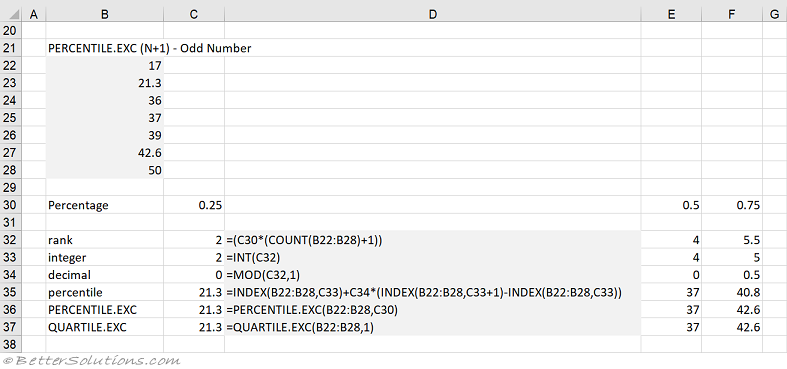# PERCENTILE.INC Function

The PERCENTILE.INC function returns the number corresponding to a particular percentage from an array of numbers.
The QUARTILE.INC function just provides a short-hand to the following values:

 QUARTILE(array,0) = PERCENTILE.INC(array,0) QUARTILE(array,1) = PERCENTILE.INC(array,0.25) QUARTILE(array,2) = PERCENTILE.INC(array,0.50) QUARTILE(array,3) = PERCENTILE.INC(array,0.75) QUARTILE(array,4) = PERCENTILE.INC(array,1)

### Analysis ToolPak

There is also Percentile functionality included in the Analysis ToolPak add-in.

### Which Method ?

There are a number of different ways to calculate a percentile value.
Excel provides two alternatives.
We will refer to them an (N-1) and (N+1).

In the (N-1) method, the ordinal rank is calculated using the formula: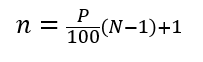In the (N+1) method, the ordinal rank is calculated using the formula: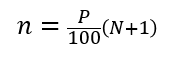Once you have the ordinal rank number the percentile can be easily calculated using the following formula
Split the ordinal rank into an integer component (k) and a decimal component (d)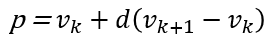### PERCENTILE.INC - N-1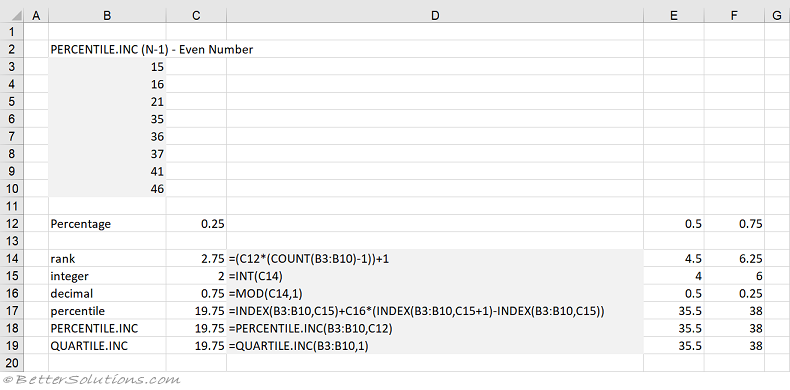### PERCENTILE.EXC - N+1